Geology - 2017 Past Year Paper

# Geology - 2017 Past Year Paper - IIT JAM

Test Description

## 60 Questions MCQ Test IIT JAM Past Year Papers and Model Test Paper (All Branches) - Geology - 2017 Past Year Paper

Geology - 2017 Past Year Paper for IIT JAM 2023 is part of IIT JAM Past Year Papers and Model Test Paper (All Branches) preparation. The Geology - 2017 Past Year Paper questions and answers have been prepared according to the IIT JAM exam syllabus.The Geology - 2017 Past Year Paper MCQs are made for IIT JAM 2023 Exam. Find important definitions, questions, notes, meanings, examples, exercises, MCQs and online tests for Geology - 2017 Past Year Paper below.
Solutions of Geology - 2017 Past Year Paper questions in English are available as part of our IIT JAM Past Year Papers and Model Test Paper (All Branches) for IIT JAM & Geology - 2017 Past Year Paper solutions in Hindi for IIT JAM Past Year Papers and Model Test Paper (All Branches) course. Download more important topics, notes, lectures and mock test series for IIT JAM Exam by signing up for free. Attempt Geology - 2017 Past Year Paper | 60 questions in 180 minutes | Mock test for IIT JAM preparation | Free important questions MCQ to study IIT JAM Past Year Papers and Model Test Paper (All Branches) for IIT JAM Exam | Download free PDF with solutions
 1 Crore+ students have signed up on EduRev. Have you?
Geology - 2017 Past Year Paper - Question 1

### Which one of the following minerals exhibits luminescenes when exposed to ultravoilet light ?

Geology - 2017 Past Year Paper - Question 2

### In which one of the following mass extinction periods trilobites became extinct ?

Geology - 2017 Past Year Paper - Question 3

### En- echelon sigmoidal ‘gash’ veins indicate

Geology - 2017 Past Year Paper - Question 4

Which one of the following primary sedimentary structures is NOT used for palaeocurrent analysis?

Geology - 2017 Past Year Paper - Question 5

The age of the Patcham Formation is

Geology - 2017 Past Year Paper - Question 6

Rivers that receive water from groundwater seepage are termed as

Geology - 2017 Past Year Paper - Question 7

Conservative plate boundary is represented by

Geology - 2017 Past Year Paper - Question 8

Which one of the following prismatic crystal forms belongs to the hexagonal crystal system ?

Geology - 2017 Past Year Paper - Question 9

The characteristic rock of contact metamorphism is

Geology - 2017 Past Year Paper - Question 10

The volcanic equivalent of nepheline syenite is

Geology - 2017 Past Year Paper - Question 11

Identify the correct match between mineral- ore and its physical property.

Geology - 2017 Past Year Paper - Question 12

Match the mineral deposits in Gourp I with their Indian occurrences in Group II.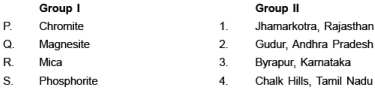Geology - 2017 Past Year Paper - Question 13

A helically coiled ammonite Turrilites is differentiated from externally resembling Gastropoda Turritella by

Geology - 2017 Past Year Paper - Question 14

The facial suture of trilobites running through the genal angle is known as

Geology - 2017 Past Year Paper - Question 15

Which one of the following statements is correct for Class 1B (Parallel) folds ?

Geology - 2017 Past Year Paper - Question 16

In the given map, the X- Y surface has the same orientation as in the Palaeozoic sequence, XY represents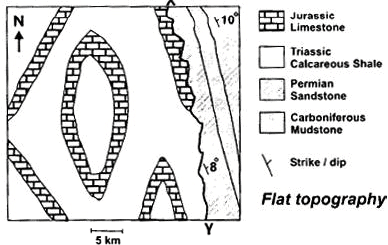Geology - 2017 Past Year Paper - Question 17

Match the sedimentary features in Group I with the corresponding sedimentary environments of their formation in Group II.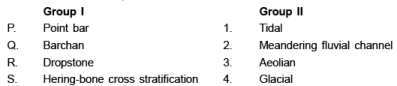Geology - 2017 Past Year Paper - Question 18

Which one of the following lithostratigraphic units id of Phanerozoic Eon ?

Geology - 2017 Past Year Paper - Question 19

Match the geological processes (Group I) with their examples in Indian stratigraphy (Group II).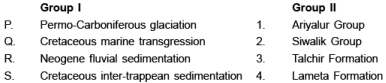Geology - 2017 Past Year Paper - Question 20

Check dams are constructed in association with main dam in the

Geology - 2017 Past Year Paper - Question 21

A geological formation neither containing not transmitting water is termed as

Geology - 2017 Past Year Paper - Question 22

Which one of the following sequences of silicate structures indicates an increasing degree of sharing of corners of (SiO4)4– tetrahedra ?

Geology - 2017 Past Year Paper - Question 23

Match the igneous bodies in Group I with their ages in Group II.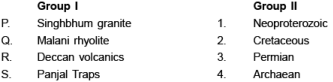Geology - 2017 Past Year Paper - Question 24

The tubefeet in echinoids emerge through

Geology - 2017 Past Year Paper - Question 25

A mineral with a point group symmetry 2/m

Geology - 2017 Past Year Paper - Question 26

The progressive metamorphic isograd that explains Barrovian metamorphism in pelite is

Geology - 2017 Past Year Paper - Question 27

In metabasic rocks, plagioclase is not stable in

Geology - 2017 Past Year Paper - Question 28

A sandstone has <5% matrix. The recalculated modal compositions of feldspar, quartz and rock fragments are 45%, 35%, 20%, respectively. The sandstone is classified as

Geology - 2017 Past Year Paper - Question 29

Match the earth layers (Group I) with corresponding approximate thicknesses (Group II).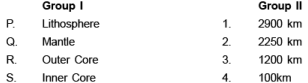Geology - 2017 Past Year Paper - Question 30

Pressure (1 GPa = 10 kbar) and temperature at the centre of the Earth are estimated to be

*Multiple options can be correct
Geology - 2017 Past Year Paper - Question 31

Choose the landsform(s) resulting from glacial erosion.

*Multiple options can be correct
Geology - 2017 Past Year Paper - Question 32

Choose the correct combination(s) of type of dentition of Bivalvia and the corresponding representative genus.

*Multiple options can be correct
Geology - 2017 Past Year Paper - Question 33

Shown below is an isobaric binary temperature- composition phase diagram in the system X- Y with complete miscibility between X and Y.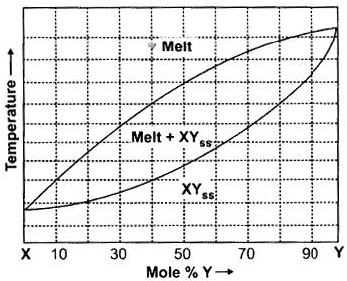W hich of the following statements is/are correct for crystallization of a starting melt of composition Z60 Y40 (the dot in the diagram) ?

*Multiple options can be correct
Geology - 2017 Past Year Paper - Question 34

Choose the correct combination(s) of textural features of magmatic rocks with corresponding petrological processes from the following.

*Multiple options can be correct
Geology - 2017 Past Year Paper - Question 35

Which of the following statements is/are NOT correct ?

*Multiple options can be correct
Geology - 2017 Past Year Paper - Question 36

In an outcrop we find that the bedding planes are vertical and cleavage surfaces are horizontal.Which of the following fold types is/are inferred from this observation ?

*Multiple options can be correct
Geology - 2017 Past Year Paper - Question 37

Which of the following stratigraphic unit(s) is/are coal/lignite bearing ?

*Multiple options can be correct
Geology - 2017 Past Year Paper - Question 38

The Toposheet No(s). immediately adjacent to Toposheet No. 55J/8 is/are

*Multiple options can be correct
Geology - 2017 Past Year Paper - Question 39

Which of the following is/are NOT true for texturally immature sandstone ?

*Multiple options can be correct
Geology - 2017 Past Year Paper - Question 40

P and S waves originate at earthquake focus and travel through the earth. Which of the following statements for these waves is/are correct ?

*Answer can only contain numeric values
Geology - 2017 Past Year Paper - Question 41

An object is spoted at S60°E front bearing from the observer. If the position is interchanged, the front bearing value in degree from North (measured clockwise) is_____.

*Answer can only contain numeric values
Geology - 2017 Past Year Paper - Question 42

The mole % of forsterite component in olivine with chemical formula Mg1.8 Fe0.2 SiO4 is _____.

*Answer can only contain numeric values
Geology - 2017 Past Year Paper - Question 43

The Weiss symbol of a crystal face is 4a: 2b: c. The value of h in the corresponding Miller Index (hkl) is _____.

*Answer can only contain numeric values
Geology - 2017 Past Year Paper - Question 44

In a mineral with chemical formula AT4 O8, the ionic radii of A and O are 1.12 Å and 1.40 Å, respectively. The co- ordination number of cation A is ____.

*Answer can only contain numeric values
Geology - 2017 Past Year Paper - Question 45

Aluminium (Al) can occur in both tetrahedral and octahedral co- ordinations in silicates. The amount of octahedral Al in a pyroxene crystal of composition MG1.4 Fe0.4 Al0.4 Si1.8 O6 is ____ (give answer in one decimal place).

*Answer can only contain numeric values
Geology - 2017 Past Year Paper - Question 46

The birefringence of a mineral of thickness 30 mm and retardation 0.27 mm is _____ (give answer in three decimal places).

*Answer can only contain numeric values
Geology - 2017 Past Year Paper - Question 47

Two limbs of a vertical chevron fold strike S70°E and N55°E. The value of the interlimb angle of the fold is ____(degree).

*Answer can only contain numeric values
Geology - 2017 Past Year Paper - Question 48

The schematic map given below shows intersecting strike lines of the same lithological contact.
In the map, AB and CD are 5cm and 3.5cm, respectively. The scale of the map is 1 cm = 100m.
The plunge of the fold axis is ____ degrees (give answer in one decimal place).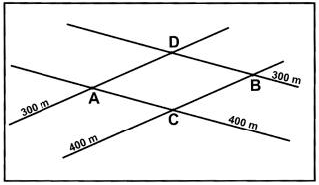*Answer can only contain numeric values
Geology - 2017 Past Year Paper - Question 49

The core- rim compositions of a normally zoned plagioclase crystal are as follows: Core: Ca0.6 Nax Al1.6 Si2.4 O8 Rim: Ca0.4 Nay Al1.4 Si2.6 O8 The amount of increase of Na atom from core to rim per formula unit of plagioclase is _____ (given answer in one decimal place).

*Answer can only contain numeric values
Geology - 2017 Past Year Paper - Question 50

Considering garnet chemical formula in 12 oxygen basis, the number of Mg cations in a garnet of chemical composition P (as shown in the figure) is ____ (give answer in two decimal places).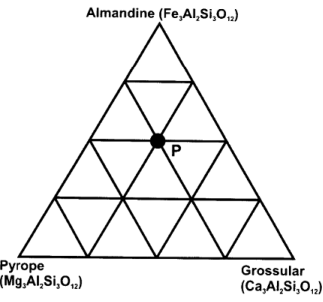*Answer can only contain numeric values
Geology - 2017 Past Year Paper - Question 51

A fault surface in an outcrop has slickenside lineation whose pitch is 30°. The horizontal slip on the fault is 1.25 m, as determined from displaced vein. The net slip on the fault is ____ meter (give answer in two decimal places).

*Answer can only contain numeric values
Geology - 2017 Past Year Paper - Question 52

In an outcrop, we find a Belemnite fossil broken into five rectangular pieces (boudins) of equal size. Long dimension of each boudin is 1.35 cm. Gap between adjacent boudins in all cases is 0.25 cm. Note that the long dimensions of boudins are perfectly aligned. The % elongation is _____ (give answer in one decimal place).

*Answer can only contain numeric values
Geology - 2017 Past Year Paper - Question 53

A horizontal cylindrical ore body (diameter = 20m, length = 200 m) has 5% metal content and density of 3500 kg/m3. The reserve of the ore body is ____ million ton(s) (give answer in two decimal places).

*Answer can only contain numeric values
Geology - 2017 Past Year Paper - Question 54

A drainage basin of fourth order covers an area of 40 sq. km. Within the basin, total length of 1st order drainage is 12.5 km, 2nd order drainage is 8.8 km, 3rd order drainage is 4.7 km and 4th order drainage is 4.0 km. The drainage density of the basin is ___ km–1 (give answer in two decimal places).

*Answer can only contain numeric values
Geology - 2017 Past Year Paper - Question 55

Age of granitic rocks can be determined using Rb- Sr whole rock radioactive dating method and the following age equation,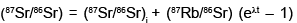For a suite of representative co- magmatic granitic rocks, the Rb- Sr whole rock isochron plot and relevant data are shown in the diagram. The age of granite is calculated at ____ Ga (1Ga = 109 yrs, give answer in one decimal place).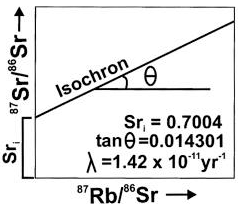*Answer can only contain numeric values
Geology - 2017 Past Year Paper - Question 56

Consider a granulite facies metamorphic rock with peak metamorphic condition at 9 kbar, 850°C.
Assume a single layer crust of ρ = 3000 kg/m3 and g = 10m/sec2 during metamorphism. The depth of burial during peak metamorphism is ____ km. 1 Pascal = 1 kg/m/sec2 and 1 bar = 105 Pascals.

*Answer can only contain numeric values
Geology - 2017 Past Year Paper - Question 57

Consider four minerals P, Q, R and S in a three component chemical system (A- B- C) as shown in the figure. For a crossing tie- line relationship, the variance (degree of freedom) of the equilibrium mineral assemblage at X is ____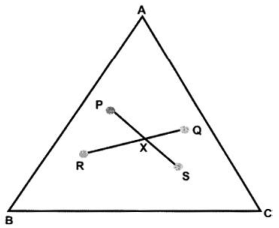*Answer can only contain numeric values
Geology - 2017 Past Year Paper - Question 58

The refractive indices of four minerals (P, Q, R, S) are as follows:

P(α = 1.712, β  = 1.721, λ  = 1.727),

Q(ε  = 1.553, ω  = 1.544),

R(α  = 1.664, β  = 1.672, λ  = 1.694) and

S(ω  = 1.658, ε  = 1.486)

The value of maximum briefringence among all the minerals is ____ (give answer in two decimal places).

*Answer can only contain numeric values
Geology - 2017 Past Year Paper - Question 59

In a sedimentary succession shown in the figure, the last occurrence of the fossil species Q (dated 50 Ma) and the first occurrence of the fossil species R (dated 30 Ma) are recorded at Y and X, respectively. The estimated rate of sedimentation is _____ m/million yrs (assume constant rate of sedimentation).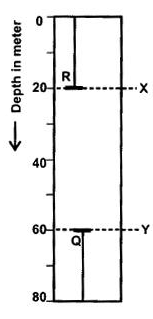*Answer can only contain numeric values
Geology - 2017 Past Year Paper - Question 60

The top surface of a coal seam is exposed at 150 m contour level on a hill top at location A.
The same surface of the seam is also exposed on a river bed at location B at the 50 m contour level. The aerial distance A- B is 1 km. The amount of dip of the coal seam along A- B is ___(degree). Give answer in one decimal place.

## IIT JAM Past Year Papers and Model Test Paper (All Branches)

8 docs|48 tests
Information about Geology - 2017 Past Year Paper Page
In this test you can find the Exam questions for Geology - 2017 Past Year Paper solved & explained in the simplest way possible. Besides giving Questions and answers for Geology - 2017 Past Year Paper, EduRev gives you an ample number of Online tests for practice

## IIT JAM Past Year Papers and Model Test Paper (All Branches)

8 docs|48 tests(Scan QR code)